# AP Calculus BC Practice Test 13

### Test Information9 questions18 minutes

Calculator Disallowed

1. Which of the following are the first four nonzero terms of the MacLaurin series for f(x) = sin(x2)?

2. The number of new members of a social media site is modeled by the function y that satisfies the differential equation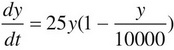, where t is the time in days and y(0) = 100. What is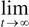y(t)?

3. For what values of x does the series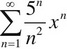converge?

4.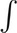xe6x dx =

5. Which of the following series converge(s)?

(I)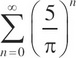(II)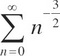(III)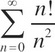6. The function f is continuous on the closed interval [0,10] and has the values in the following table: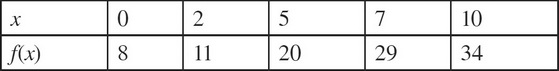Using the subintervals [0, 2], [2, 5], [5, 7], and [7, 10], approximate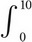f(x)dx using a left Riemann sum.

7.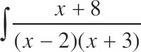dx =

8. Evaluate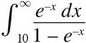9. What is the coefficient of x8 in the Taylor series for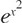about x = 0?